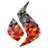22-26 March 2021

# Implementation of a nonlinear anisotropic image denoising model in FEniCS

Abderrazzak Boufala (LISTI lab-ENSA, FSJES AM, Ibn Zohr University, 🇲🇦)
El Mostafa Kalmoun (Department of Mathematics, Statistics and Physics, College of Arts and Sciences, Qatar University, 🇶🇦)
Thursday session 2 (Zoom) (15:00–16:30 GMT)
10.6084/m9.figshare.14495499

In this talk, we present a numerical implementation of the following nonlinear anisotropic diffusion-based image denoising model, using the computing platformFEniCS Project:

$$u-u_{0} = \frac{1}{2 \lambda} \operatorname{div} \left(\frac{1}{\left(\epsilon^2+\vert \nabla u_\sigma \vert^2\right)^{1-p/2}}\nabla u \right) \qquad \text{in } \Omega,$$

$$\partial_n u = 0 \qquad \text{on } \partial\Omega.$$

$$u=u(x,y)$$ denotes the unknown image to be recovered, $$u_{0}$$ is the observed noisy image, $$\Omega \subset \mathbb{R}^{2}$$ is the spatial image domain and $$\partial_{n} u$$ denotes the derivative of $$u$$ in the direction normal to the boundary $$\partial \Omega$$.

We also compare the numerical results with those obtained using finite difference method.